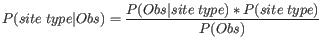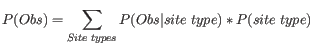## Calculation of the posterior probability for each site type at each position in the genome

Based on the probabilities calculated, one can determine which of the site types is the best fit at each position in the genome. The site type determined to be the most likely at each position can then be compared with the allele in the reference sequence at the same position. If it is likely to be different, it suggests the presence of a variation.

Therefore the posterior probability is formed as follows:where